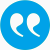#Piribauer, Philipp, Fischer, Manfred M. 2015. Model uncertainty in matrix exponential spatial growth regression models. Geographical Analysis. An International Journal of Theoretical Geography 47 (3), 240-261.

BibTeX

## Abstract

This paper considers the most important aspects of model uncertainty for spatial regression models, namely the appropriate spatial weight matrix to be employed and the appropriate explanatory vari- ables. We focus on the spatial Durbin model (SDM) specification in this study that nests most models used in the regional growth literature, and develop a simple Bayesian model averaging approach that provides a unified and formal treatment of these aspects of model uncertainty for SDM growth models. The approach expands on the work by LeSage and Fischer (2008) by reducing the computational costs through the use of Bayesian information criterion model weights and a matrix exponential specification of the SDM model. The spatial Durbin matrix exponential model has theoretical and computational advantages over the spatial autoregressive specification due to the ease of inversion, differentiation and integration of the matrix expo- nential. In particular, the matrix exponential has a simple matrix determinant which vanishes for the case of a spatial weight matrix with a trace of zero (LeSage and Pace 2007). This allows for a larger domain of spatial growth regression models to be analysed with this approach, including models based on different classes of spatial weight matrices. The working of the approach is illustrated for the case of 32 potential determinants and three classes of spatial weight matrices (contiguity-based, k-nearest neighbor and distance-based spatial weight matrices), using a dataset of income per capita growth for 273 European regions. (authors' abstract)

## Tags

Press 'enter' for creating the tag

## Publication's profile

Status of publication Published WU Journal article Geographical Analysis. An International Journal of Theoretical Geography SSCI A English Model uncertainty in matrix exponential spatial growth regression models 47 3 2015 240 261 http://dx.doi.org/10.1111/gean.12057

## Associations

People
Piribauer, Philipp (Former researcher)
Fischer, Manfred M. (Details)
Organization
Research Institute for Supply Chain Management FI (Details)
Institute for Economic Geography and GIScience IN (Details)